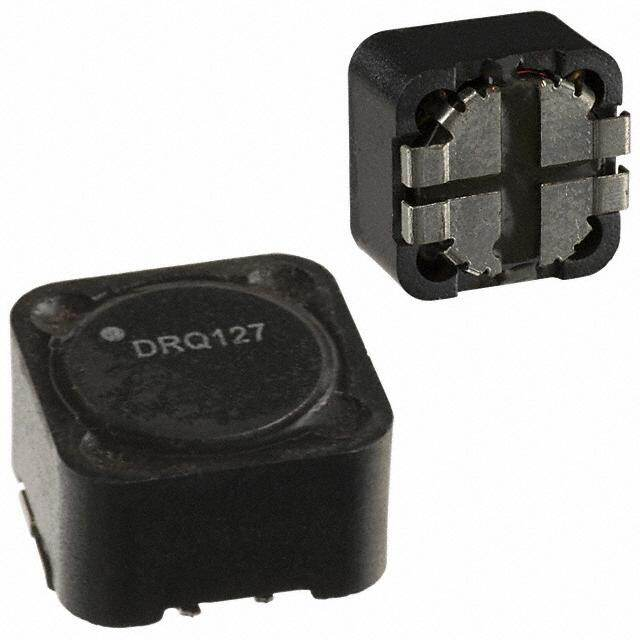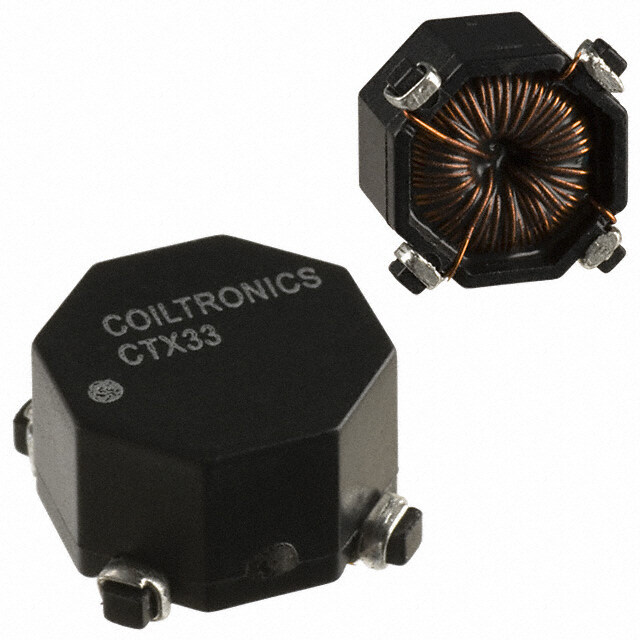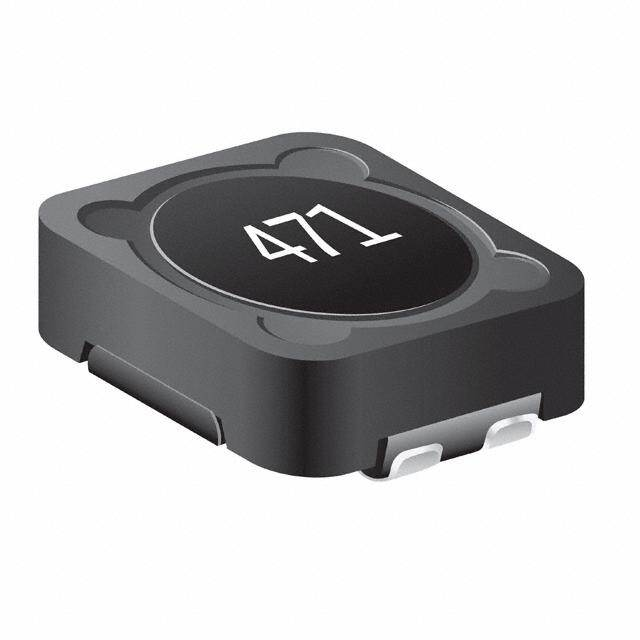RoHSx

1DRQ127-331-R Eaton - Electronics Division Y Shielded 2 Coil Inductor Array 1.294mH Inductance - Connected in Series 323.6μH Inductance - Connected in Parallel 574mOhm DC Resistance (DCR) - Parallel 1.04A Nonstandard
2DRQ127-3R3-R Eaton - Electronics Division Y Shielded 2 Coil Inductor Array 11.32μH Inductance - Connected in Series 2.831μH Inductance - Connected in Parallel 5.67mOhm DC Resistance (DCR) - Parallel 10.4A Nonstandard
3DRQ127-330-R Eaton - Electronics Division Y Shielded 2 Coil Inductor Array 135.7μH Inductance - Connected in Series 33.92μH Inductance - Connected in Parallel 60mOhm DC Resistance (DCR) - Parallel 3.23A Nonstandard
4DRQ127-3R3-R Eaton - Electronics Division Y Shielded 2 Coil Inductor Array 11.32μH Inductance - Connected in Series 2.831μH Inductance - Connected in Parallel 5.67mOhm DC Resistance (DCR) - Parallel 10.4A Nonstandard
5DRQ127-330-R Eaton - Electronics Division Y Shielded 2 Coil Inductor Array 135.7μH Inductance - Connected in Series 33.92μH Inductance - Connected in Parallel 60mOhm DC Resistance (DCR) - Parallel 3.23A Nonstandard
6DRQ127-331-R Eaton - Electronics Division Y Shielded 2 Coil Inductor Array 1.294mH Inductance - Connected in Series 323.6μH Inductance - Connected in Parallel 574mOhm DC Resistance (DCR) - Parallel 1.04A Nonstandard
7DRQ127-3R3-R Eaton - Electronics Division Y Shielded 2 Coil Inductor Array 11.32μH Inductance - Connected in Series 2.831μH Inductance - Connected in Parallel 5.67mOhm DC Resistance (DCR) - Parallel 10.4A Nonstandard
8DRQ127-330-R Eaton - Electronics Division Y Shielded 2 Coil Inductor Array 135.7μH Inductance - Connected in Series 33.92μH Inductance - Connected in Parallel 60mOhm DC Resistance (DCR) - Parallel 3.23A Nonstandard
9DRQ127-331-R Eaton - Electronics Division Y Shielded 2 Coil Inductor Array 1.294mH Inductance - Connected in Series 323.6μH Inductance - Connected in Parallel 574mOhm DC Resistance (DCR) - Parallel 1.04A Nonstandard
10DRQ127-330-R Eaton - Electronics Division Y Shielded 2 Coil Inductor Array 135.7μH Inductance - Connected in Series 33.92μH Inductance - Connected in Parallel 60mOhm DC Resistance (DCR) - Parallel 3.23A Nonstandard
11DRQ127-330-R Eaton - Electronics Division Y Shielded 2 Coil Inductor Array 135.7μH Inductance - Connected in Series 33.92μH Inductance - Connected in Parallel 60mOhm DC Resistance (DCR) - Parallel 3.23A Nonstandard
12DRQ127-330-R Eaton - Electronics Division Y Shielded 2 Coil Inductor Array 135.7μH Inductance - Connected in Series 33.92μH Inductance - Connected in Parallel 60mOhm DC Resistance (DCR) - Parallel 3.23A Nonstandard
13CTX33-2P-R Eaton - Electronics Division Y Unshielded 2 Coil Inductor Array 130.7μH Inductance - Connected in Series 32.67μH Inductance - Connected in Parallel 126mOhm Max DC Resistance (DCR) - Parallel 1.3A Nonstandard
14CTX33-2P-R Eaton - Electronics Division Y Unshielded 2 Coil Inductor Array 130.7μH Inductance - Connected in Series 32.67μH Inductance - Connected in Parallel 126mOhm Max DC Resistance (DCR) - Parallel 1.3A Nonstandard
15CTX33-2P-R Eaton - Electronics Division Y Unshielded 2 Coil Inductor Array 130.7μH Inductance - Connected in Series 32.67μH Inductance - Connected in Parallel 126mOhm Max DC Resistance (DCR) - Parallel 1.3A Nonstandard
16CTX33-2P-R Eaton - Electronics Division Y Unshielded 2 Coil Inductor Array 130.7μH Inductance - Connected in Series 32.67μH Inductance - Connected in Parallel 126mOhm Max DC Resistance (DCR) - Parallel 1.3A Nonstandard
17CTX33-2P-R Eaton - Electronics Division Y Unshielded 2 Coil Inductor Array 130.7μH Inductance - Connected in Series 32.67μH Inductance - Connected in Parallel 126mOhm Max DC Resistance (DCR) - Parallel 1.3A Nonstandard
18CTX33-2P-R Eaton - Electronics Division Y Unshielded 2 Coil Inductor Array 130.7μH Inductance - Connected in Series 32.67μH Inductance - Connected in Parallel 126mOhm Max DC Resistance (DCR) - Parallel 1.3A Nonstandard
19SRF0703-220M Bourns Inc. Y Shielded 2 Coil Inductor Array 88μH Inductance - Connected in Series 22μH Inductance - Connected in Parallel 150mOhm Max DC Resistance (DCR) - Parallel 1.62A Nonstandard
20SRF0703-220M Bourns Inc. Y Shielded 2 Coil Inductor Array 88μH Inductance - Connected in Series 22μH Inductance - Connected in Parallel 150mOhm Max DC Resistance (DCR) - Parallel 1.62A Nonstandard

### 還有更多產品分類沒有全部展示，請重新設置過濾條件篩選

手機、郵箱任意一項必填，公司、聯系人選填

yo飞禽走兽多人版